# The Triaugmented Dodecahedron

The triaugmented dodecahedron (J61) is the 61st among the Johnson solids. It has 23 vertices, 45 edges, and 24 faces (9 pentagons and 15 triangles). It is constructed by augmenting a dodecahedron with 3 pentagonal pyramids on non-adjacent pentagonal faces.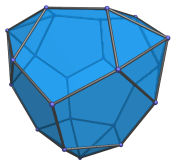If one of the pentagonal pyramids is removed, the result is the metabiaugmented dodecahedron (J60). If two pentagonal pyramids are removed, the result is the augmented dodecahedron (J58).

## Projections

Here are some views of the triaugmented dodecahedron from various angles:

Projection Description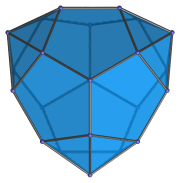Top view.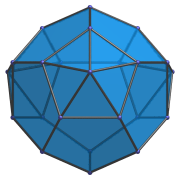Front view.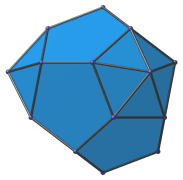Side view, parallel to pair of opposite pentagons.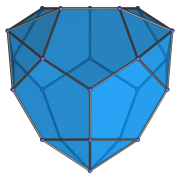Parallel to pentagon opposite an augment.

## Coordinates

The Cartesian coordinates of the triaugmented dodecahedron, centered on the origin and with edge length 2, are:

• (0, ±φ2, ±1)
• (±1, 0, ±φ2)
• (±φ2, ±1, 0)
• (±φ, ±φ, ±φ)
• (0, (7+φ)/5, ±(8φ+1)/5)
• ((7+φ)/5, −(8φ+1)/5, 0)

where φ=(1+√5)/2 is the Golden Ratio.

Last updated 16 Jan 2019.